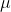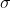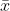##### Question

In: Statistics and Probability

# Salaries for teachers in a particular elementary school district are normally distributed with a mean of...

Salaries for teachers in a particular elementary school district are normally distributed with a mean of $46,000 and a standard deviation of$4,500. We randomly survey ten teachers from that district. (Round your answers to the nearest dollar.)

A) Find the 90th percentile for an individual teacher's salary.

B)Find the 90th percentile for the average teacher's salary.

## Solutions

##### Expert Solution

Solution:

Given that,

mean == 46,000

standard deviation == 4,500

Using standard normal table,

a ) P(Z < z) = 90%

P(Z < z) = 0.90

P(Z < 1.28) = 0.27

z = 1.28

Using z-score formula,

x = z *+x = 1.28 * 4,500 + 46,000

x = 51760

The 90th percentile =51760

b )n = 10= 46,000=/ n = 4,50010 =1423.0249

P(Z < z) = 90%

P(Z < z) = 0.90

P(Z < 1.28) = 0.27

z = 1.28

Using z-score formula,= z *+= 1.28 * 1423.0249 + 46,000= 47821.47

The 90th percentile =47821

## Related Solutions

##### Salaries for teachers in a particular elementary school district are normally distributed with a mean of...
Salaries for teachers in a particular elementary school district are normally distributed with a mean of $44,000 and a standard deviation of$6,500. We randomly survey ten teachers from that district. 1.Find the probability that the teachers earn a total of over $400,000 2.If we surveyed 70 teachers instead of ten, graphically, how would that change the distribution in part d? 3.If each of the 70 teachers received a$3,000 raise, graphically, how would that change the distribution in part...
##### Salaries for teachers in a particular elementary school district are normally distributed with a mean of...
Salaries for teachers in a particular elementary school district are normally distributed with a mean of $44,000 and a standard deviation of$6,500. We randomly survey ten teachers from that district. Find the 85th percentile for the sum of the sampled teacher's salaries to 2 decimal places.
##### A researcher claims that the mean of the salaries of elementary school teachers is greater than...
A researcher claims that the mean of the salaries of elementary school teachers is greater than the mean of the salaries of secondary school teachers in a large school district. The mean of the salaries of a random sample of 26 elementary school teachers is $48,250 and the sample standard deviation is$3900. The mean of the salaries of 24 randomly selected secondary school teachers is $45,630 with a sample standard deviation of$5530. At ? = 0.05, can it...
##### A study was conducted to estimate the difference in the mean salaries of elementary school teachers...
A study was conducted to estimate the difference in the mean salaries of elementary school teachers from two neighboring states. A sample of 10 teachers from the Indiana had a mean salary of $28,900 with a standard deviation of$2300. A sample of 14 teachers from Michigan had a mean salary of $30,300 with a standard deviation of$2100. Determine a 95% confidence interval for the difference between the mean salary in Indiana and Michigan.(Assume population variances are different.) *Include...
##### A researcher claims that the variation in the salaries of elementary school teachers is greater than...
A researcher claims that the variation in the salaries of elementary school teachers is greater than the variation in the salaries of secondary school teachers. A random sample of the salaries of 30 elementary school teachers has a variance of $8,208, and a random sample of the salaries of 30 secondary school teachers has a variance of$3,817. At α = 0.05, can the researcher conclude that the variation in the elementary school teachers’ salaries is greater than the variation...